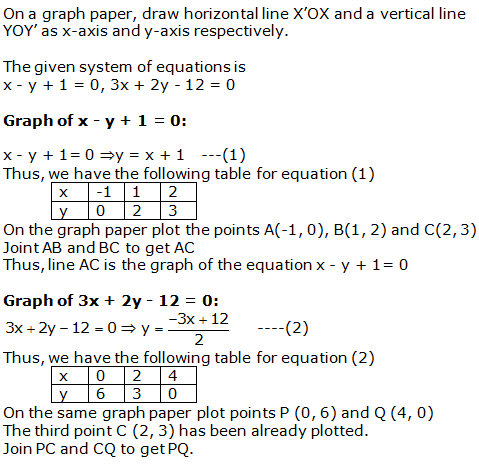# R S AGGARWAL AND V AGGARWAL Solutions for Class 10 Maths Chapter 3 – Linear equations in two variables

## Chapter 3 – Linear equations in two variables Exercise Ex. 3A

Question 1

Solve each of the following systems of equations graphically:

2x + 3y = 2

x – 2y = 8

Solution 1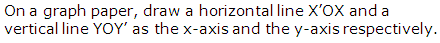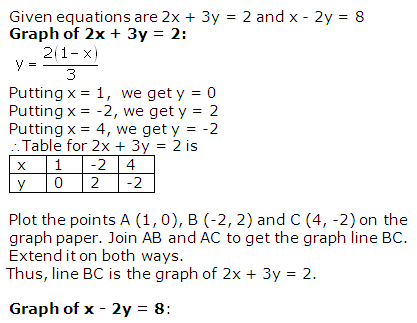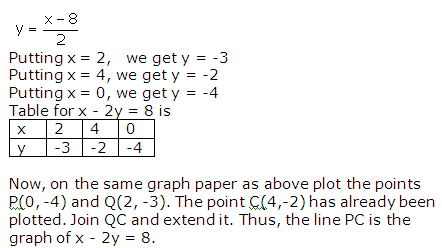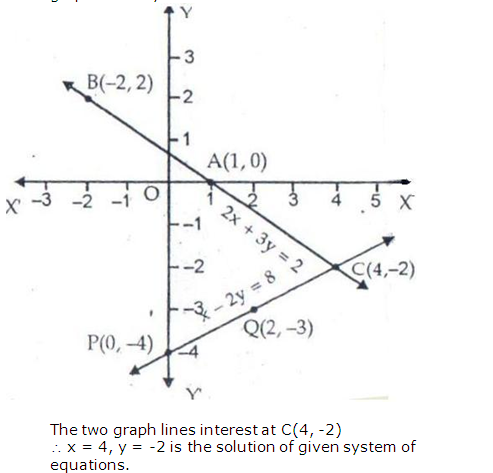Question 2

Solve each of the following systems of equations graphically:

3x + 2y = 4

2x – 3y = 7

Solution 2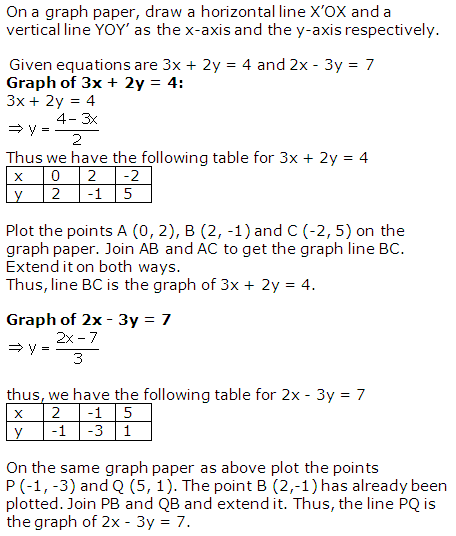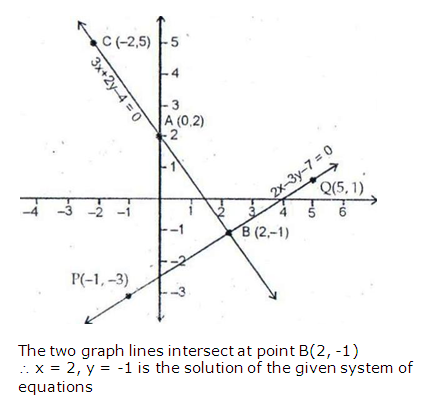Question 3

Solve each of the following systems of equations graphically:

2x + 3y = 8

x – 2y + 3 = 0

Solution 3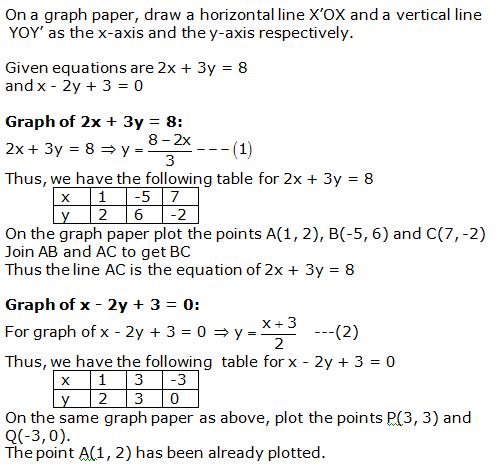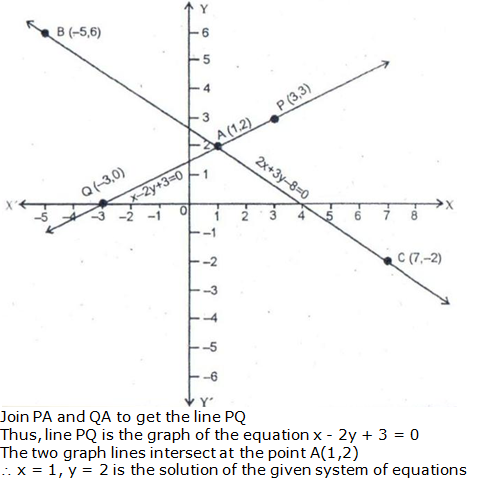Question 4

Solve each of the following systems of equations graphically:

2x – 5y + 4 = 0

2x + y – 8 = 0

Solution 4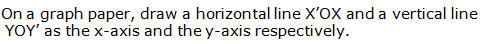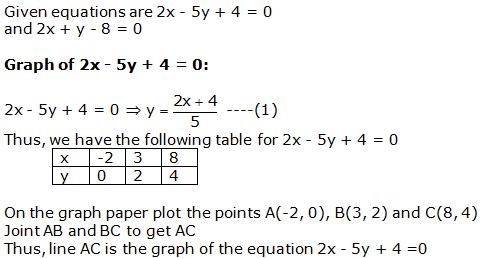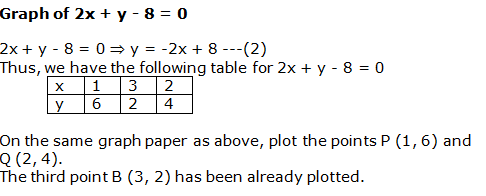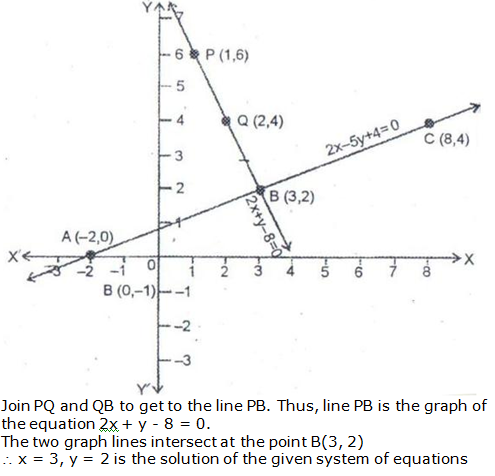Question 5

Solve
each of the following systems of equations graphically:

3x
+ 2y = 12, 5x – 2y = 4.

Solution 5Since the two graphs intersect at (2, 3),

x = 2 and y = 3.

Question 6

Solve each of the following systems of equations graphically:

3x + y + 1 = 0

2x – 3y + 8 = 0

Solution 6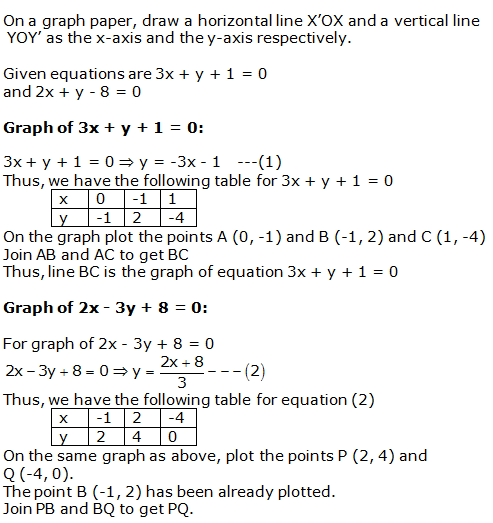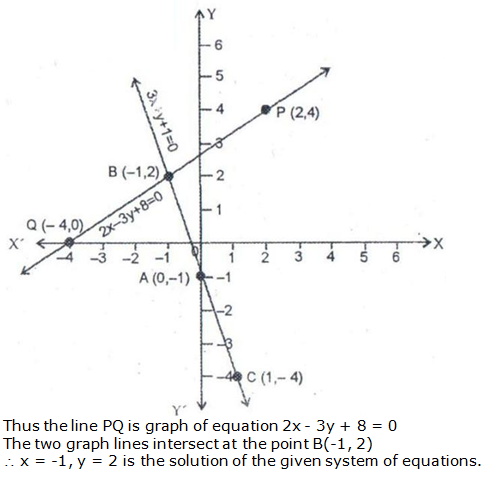Question 7

Solve
each of the following systems of equations graphically:

2x
+ 3y + 5 = 0, 3x – 2y – 12 = 0.

Solution 7Since the two graphs intersect at (2, -3),

x = 2 and y = -3.

Question 8

Solve
each of the following systems of equations graphically:

2x
– 3y + 13 = 0, 3x – 2y + 12 = 0.

Solution 8Since the two graphs intersect at (-2, 3),

x = -2 and y = 3.

Question 9

Solve
each of the following systems of equations graphically:

2x
+ 3y – 4 = 0, 3x – y + 5 = 0.

Solution 9Since the two graphs intersect at (-1, 2),

x = -1 and y = 2.

Question 10

Solve each of the following systems of equations graphically:

x + 2y + 2 = 0

3x + 2y – 2 = 0

Solution 10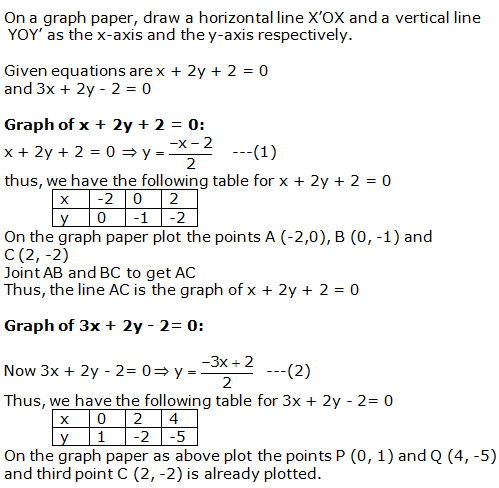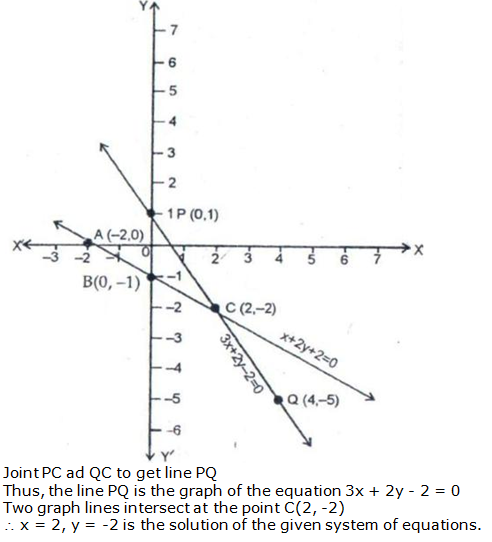Question 11

Solve
each of the following given systems of equations graphically and find the
vertices and area of the triangle formed by these lines and the x-axis:

x
y + 3 = 0, 2x + 3y – 4 = 0.

Solution 11Question 12

Solve
each of the following given systems of equations graphically and find the
vertices and area of the triangle formed by these lines and the x-axis:

2x
– 3y + 4 = 0, x + 2y – 5 = 0.

Solution 12Question 13

Solve the following system of linear equations graphically:

4x – 3y + 4 = 0, 4x + 3y – 20 = 0

Find the area of the region bounded by these lines and the x-axis.

Solution 13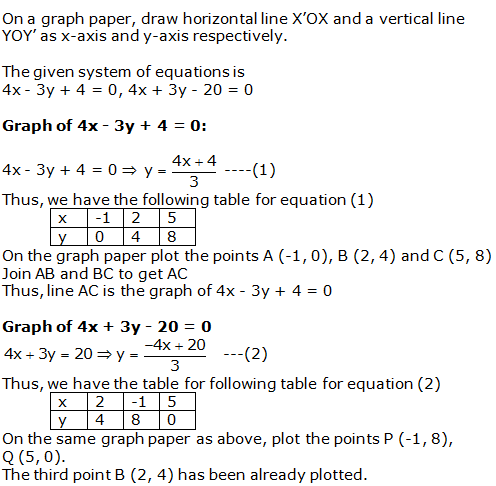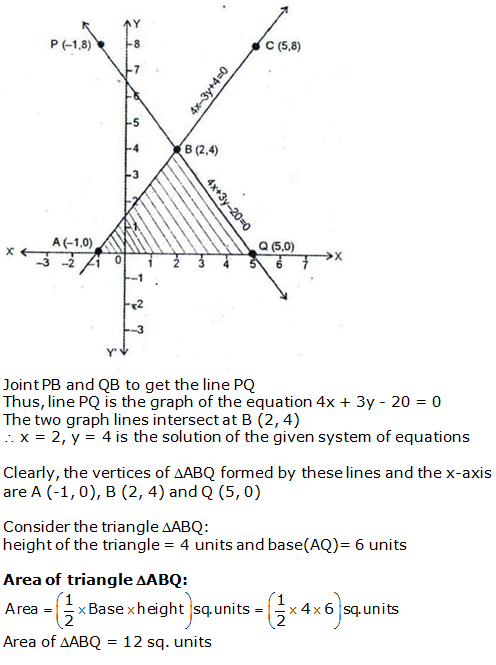Question 14

Solve the following system of linear equation graphically:

x – y + 1 = 0, 3x + 2y – 12 = 0

Calculate the area bounded by these lines and x-axis.

Solution 14